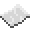# math.acos

Return the arccos (inverse cosine) of num (in radians). This is equivalent to $\arccos(x)$. To convert the output to degrees, you can use math.deg (for example: math.deg(math.acos(0.5))).

Name arccos math.acos $y=\arccos(x)$$x=\cos(y)$$-1\leq x\leq 1$$0\leq y\leq \pi$ExamplePrint the arccosine of user input
Read a line from the user, print its arccos in radians, then in degrees.
Code
<nowiki>local num = math.acos(tonumber(read()))
print(num)
print(math.deg(num))
</nowiki>

Output Depends on what the user wrote. For instance, if they were to enter 0.5, the numbers printed would be 1.0471975512 (${\frac {\pi }{3}}$) and 60.

math.acos
Function
Syntax
math.acos(
• num : number
)

Returns number
API math
Source Lua (source)